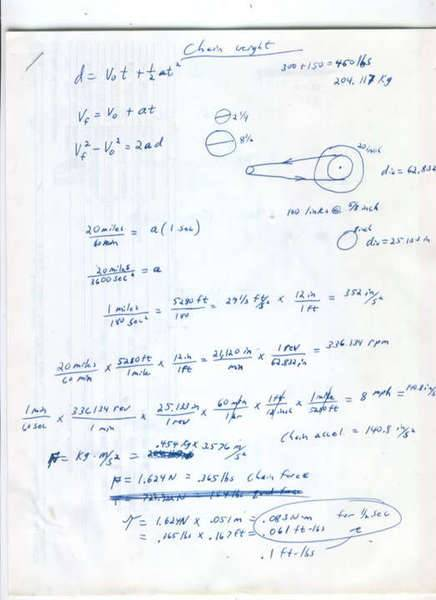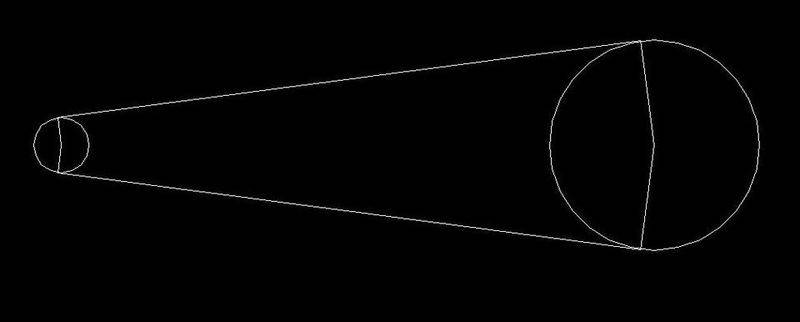# Power needed to accelerate a chain.

I'm not a student, but this seemed like the correct place to put a question.

I need to know how much power it takes to accelerate a motorcycle chain weighing 1 pound in 1 second to a speed that would equal 20mph at the wheel from a dead stop.

The wheel has a diameter of 20 inches.
The drive sprocket has a diameter of 2.25 inches.
The driven sprocket has a diameter of 8.5 inches.
If for some reason we need the length of the chain, I suppose that would be 100 links at 5/8 inch per link.
Assume all frictions are zero and all sprockets and wheels are massless.

Here's where I tried:## Answers and Replies

I am having sort of a hard time following your calculations. The problem itself isn't difficult but a bit tricky because of the chain revolving around the sprockets.

Power = F x Vchain

The way I would attack this problem is to split the chain up into 3 pieces. One piece being the two strait piece of chain moving at a strait velocity. The other two pieces being half (approximately) of each sprocket. The first part will simply be:

Ps = (Mc*a) x (Vo*t + .5*a*t^2)

However for the sprockets you need to consider the moment of gyration or rotational moment of inertia in order to determine the torque that needs to be placed on the chain. The chain can be modeled as a simple ring.

Ig = (Mc*r1^2)/2 + (Mc*r2^2)/2

Where the power is:

Pc = (Ig*alpha) x (wo*t + .5*alpha*t^2)

So your total power would be:

Pt = Ps + Pc

Last edited:
Nevermind my scribblings... I think I was barking up the wrong tree anyway. :D

You make it look pretty straight forward. The only thing I don't understand is alpha and wo???

So, if the tire is spinning at 20 mph, then (20 miles / 60 min) * (5280 ft / 1 mile) * (12 in / 1 ft) * (1 revolution / 62.832 in circumference) = 336.134 rpm

If the driven sprocket is turning 336.134 rpm, then (1 min / 60 sec) * (336.134 rev / 1 min) * (26.7035 in sprocket circumference / 1 min) = 149.599 in/s chain velocity.

Since Vf=Vo+at, then 149.599 in/s = 0 + a(1 second).... Solve for a = 149.599 in/s/s

Then 149.599 in = 3.7998 m

Then Ps = (.4536Kg * 3.7998 m/s/s) * (0 + .5 * 3.7998 m/s/s * (1 s) * (1 s)) = 3.2746 Nm (but that's torque, not power????)

Am I right so far?

but that's torque, not power????

Thats because I screwed up and gave you the wrong equation, what you calculated was the work not the power. Sorry about that, guess it was to early in the day for me.

It should be P = F x V, So;

P = (Mc * a) x (a*t)

Alpha is the angular acceleration, and wo is initial angular velocity. Basically the equations as whats used for the strait part of the chain but with a rotational motion and Euler's equation.

Well, if that's the worst you do on a bad day, you must be a REAL wizard once you get warmed up!

Anyway, so, Ps = (.4536Kg * 3.7998 m/s/s) * (3.7998 m/s/s * 1 second) = 6.5493 Nm/s Ok, that looks like power, lol.

Wait... Would the mass Mc be the mass ONLY of the part of the chain going straight? Seems like it would be... I think I screwed up. And likewise the mass of the chain traveling ONLY around the sprocket? And to complicate things, the chain doesn't wrap a full 180 degrees around the sprocket either... Do you think it matters much?

Like this:Wait... Would the mass Mc be the mass ONLY of the part of the chain going straight? Seems like it would be... I think I screwed up. And likewise the mass of the chain traveling ONLY around the sprocket? And to complicate things, the chain doesn't wrap a full 180 degrees around the sprocket either... Do you think it matters much?

You are correct. You only want to use the mass of the two sections that are going strait. Whether or not you assume the chains wrap 180 or not is up to and how accurate you want your calculations to be. Since your not considering friction I would just assume they wrap 180 degrees around the sprocket. That was the assumption I made in post #2

The other two pieces being half (approximately) of each sprocket.

I'm confused about the angular acceleration. Wouldn't that be the same as the linear acceleration since everything is connected?

Since P is constant,

E = P t
E = (mv^2)/2
P = (mv^2)/2t

m = 1 pound = .45 kg
v = 20 mph = 20 * 1609 m / 3600 s = 8.9 m/s
t = 1 sec

E = 17.8 J
P = 17.8 W

My error. The speed of the chain is 8.5 mph not 20, because of the ratio of the driven sprocket to the wheel diameter. So multiply my previous answer by (8.5/20)^2.

P= 3.2 W

So, is it just that easy? Since the chain is connected we can treat it all as if it were going in a straight line??? We don't need this moment of inertia stuff? Because I was starting to get some weird figures using those other equations:

Ig = (Mc*r1^2)/2 + (Mc*r2^2)/2
Pc = (Ig*alpha) x (alpha*t)

Ig = (.0235Kg * .0286m * .0286m) * 164/360 + (.1058Kg * .1080m * .1080m) * 196/360 = .00068063Kgmm

Pc = (.00068063Kgmm * 3.7998 m/s/s) * (3.7998 m/s/s * 1 second) = Huh???

f you consider the bike moving and ignore the turning of the chain, the energy added is 17.8 J. This is easy because the bike is moving at 20 mph.

(this is the calculation in my first reply)

If you consider the chain turning, but the bike not moving, the energy added from rest is just 3.2 J. This is easy because every part of the chain is moving at 8.5 mph.

(my second reply)

You can just add these to get the total energy which is 21 J which requires a power of 21 W for 1 second.

Well, all I'm really getting at here is whether I should spend more money to buy a chain that weighs about 1/2 a pound less than a cheaper chain.

The bike is not moving.... The idea is to just break the tires loose and slide. 3J doesn't sound like much. The engine has around 20HP.